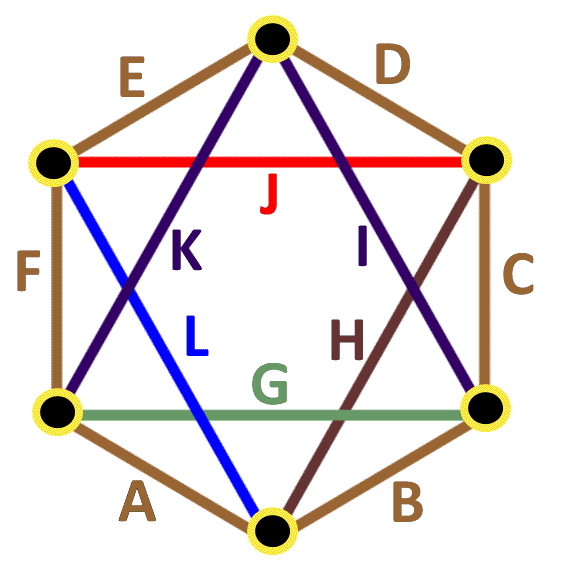BrainDen.com - Brain Teasers
• 0

# Equal product of dots in Hexagon

## Question

Put number 2,3,5,6,10,12,15,18,20,45,50, and 75 to each line in the picture, so the product of lines which meet in every dot are equal.

A*L*H*B = B*G*I*C = C*H*J*D = D*I*K*E = E*J*L*F = F*K*G*A

if rotation and reflection are considered as 1 same solution, There are 16 solutions.## Recommended Posts

• 0
Spoiler

For what it's worth, if rotation and reflection are NOT considered one solution, then there are 196 answers...

Also, interestingly, all solutions have a product of 27,000.

Here's one configuration that works:

a=2, b=6, c=3, d=15, e=5, f=10, g=75, h=50, i=20, j=12, k=18, l=45

## Join the conversation

You can post now and register later. If you have an account, sign in now to post with your account.×   Pasted as rich text.   Paste as plain text instead

Only 75 emoji are allowed.

×   Your previous content has been restored.   Clear editor

×   You cannot paste images directly. Upload or insert images from URL.